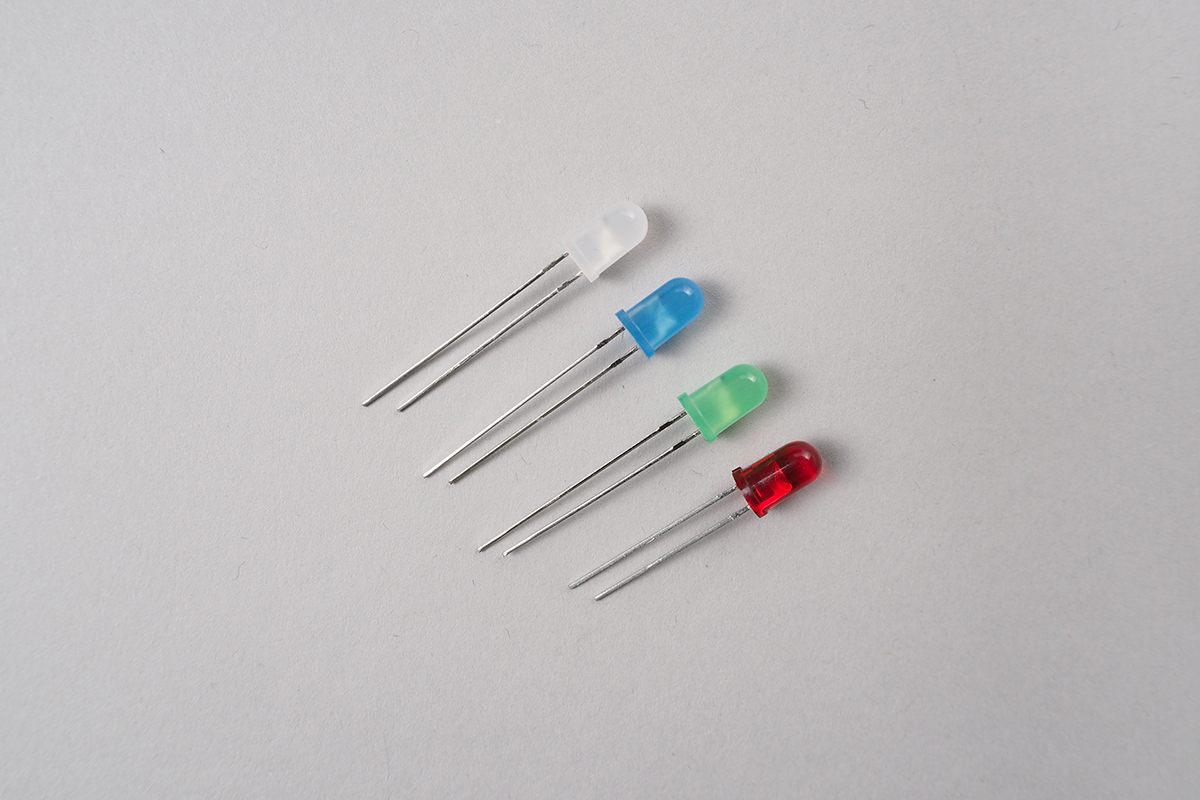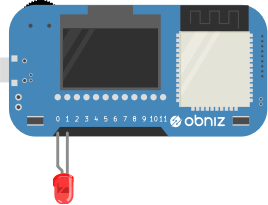# LED# LED

LED bright by supplying voltage.
It has two pins anode and cathode.
connect anode to plus and cathode to minus then it bright.
anode is a little bit longer than cathode.## obniz.wired("LED", {anode, cathode})

anode is loger leg.
specify obniz Board io numbers you connected LED.

You need a resistor if you are not using a resistor embeded LED.``````// Javascript Example
var led = obniz.wired("LED", {anode:0, cathode:1}); // io0 is connected to anode, io1 is cathode
led.on();
``````
``````// Javascript Example
var led = obniz.wired("LED", {anode:0}); // io0 is anode. cathode is connected obniz GND other way.
led.on();
``````

## on()

Simply, Turning on a LED.
It provide 5V to LED.

``````// Javascript Example
var led = obniz.wired("LED", {anode:0, cathode:1});
led.on();
``````

## off()

Turning off a LED.

``````// Javascript Example
var led = obniz.wired("LED", {anode:0, cathode:1});
led.off();
``````

## output(value)

on, off a LED regarding value.

``````// Javascript Example
var led = obniz.wired("LED", {anode:0, cathode:1});
led.output(true);
``````

default interval is 100msec

``````// Javascript Example
var led = obniz.wired("LED", {anode:0, cathode:1});
``````// Javascript Example